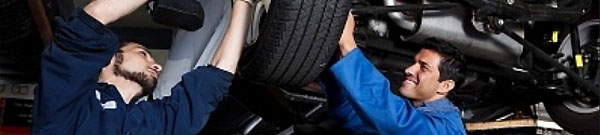# Principle of Production of Exhaust GasesTheoretical Air-fuel Ratio

The theoretical air-fuel ratio is the ratio of the minimum amount of fuel and air (which contains oxygen) needed to fully burn the fuel. Gasoline is a mixture of several types of hydrocarbons of which is the most predominant octane (C8H18). 2C8H18 + 25O2 !16CO2 + 18H2O In order for 1g of octane to produce water (H2O) and carbon dioxide (CO2) when burned, 15g of air is necessary. The actual fuel is not pure octane but octane and various HCs. Therefore the theoretical air-fuel ratio is about 14.7.

Graph Showing the Generation of CO/HC/NOx

The graph on the left, shows the air-fuel ratio and theamounts of CO/HC/NOx generated.

1. Richer

CO/HCIncrease

NOxDecrease

2. Leaner

CODecrease

HCDecrease

However it increases because of the misfire when the air-fuel ratio is too lean. NOxThe amount generated is greatest when the actual air-fuel ratio is slightly leaner than the theoretical air-fuel ratio. When the ratio becomes even leaner, the amount decreases because the combustion temperature decreases. The generated amounts of CO/HC/NOx increase under the following conditions, except for the graph on the left.

3. While engine is cold

The generated amounts of CO/HC increase because the rich air-fuel mixture is supplied.